# NEET Physics Electromagnetic Waves Questions Solved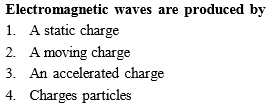Concept Videos :-

#6 | Production of EM Wave

Concept Questions :-

Generation of EM waves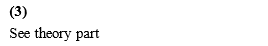Difficulty Level:

• 7%
• 17%
• 70%
• 8%
Crack NEET with Online Course - Free Trial (Offer Valid Till September 19, 2019)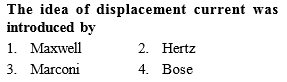Concept Videos :-

#4 | Logical Inconsistency in Ampere's Circuital Law

Concept Questions :-

Problem with Ampere's law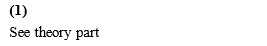Difficulty Level:

• 84%
• 11%
• 6%
• 1%
Crack NEET with Online Course - Free Trial (Offer Valid Till September 19, 2019)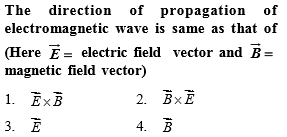Concept Videos :-

#7 | What is EM Wave?
#8 | Transverse Nature of EM Wave
#9 | Properties (1 to 9) of EM Wave
#10 | Properties (10, 11, 12) of EM Wave
#11 | Spectrum of EM Waves
#12 | Solved Examples (1 to 5)
#13 | Solved Examples (6 to 9)

Concept Questions :-

Properties of EM waves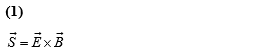Difficulty Level:

• 80%
• 15%
• 4%
• 3%
Crack NEET with Online Course - Free Trial (Offer Valid Till September 19, 2019)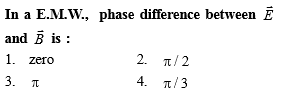Concept Videos :-

#6 | Production of EM Wave

Concept Questions :-

Generation of EM waves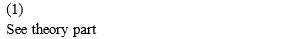Difficulty Level:

• 44%
• 48%
• 7%
• 3%
Crack NEET with Online Course - Free Trial (Offer Valid Till September 19, 2019)
NEET - 2017

In an electromagnetic wave in free space the root mean square value of the electric field is ${E}_{rms}=6$ V/m. The peak value of the magnetic field is

(a) 1.41$×{10}^{-8}T$

(b) $2.83×{10}^{-8}T$

(c) $0.70×{10}^{-8}T$

(d)

Concept Videos :-

#7 | What is EM Wave?
#8 | Transverse Nature of EM Wave
#9 | Properties (1 to 9) of EM Wave
#10 | Properties (10, 11, 12) of EM Wave
#11 | Spectrum of EM Waves
#12 | Solved Examples (1 to 5)
#13 | Solved Examples (6 to 9)

Concept Questions :-

Properties of EM waves

(b) Given root mean square value of electric field,

We know that, peak value of electric field,

${E}_{o}=\sqrt{2}{E}_{rms}$

Also, we know that, $c=\frac{{E}_{o}}{{B}_{o}}$

where, c= speed of light in vacuum

Difficulty Level:

• 17%
• 60%
• 14%
• 11%
Crack NEET with Online Course - Free Trial (Offer Valid Till September 19, 2019)
NEET - 2016

Out of the following options which one can be used to produce a propagating electromagnetic wave?

(a) A stationary charge               (b) A chargeless particle

(c) An accelerating charge          (d) A charge moving at constant velocity

Concept Videos :-

#6 | Production of EM Wave

Concept Questions :-

Generation of EM waves

(c) An electric charge at rest has electric field in the region around it, but no magnetic field. A moving charge produces both the electric and magnetic fields. If a charge is moving with a constant velocity, the electric and magnetic fields will not change with time, hence no EM wave will be produced. But if the charge is moving with a non-zero acceleration, both the electric and magnetic field will change will space and time, it then produced EM wave. This shows that accelerated charge emits electromagnetic waves.

Difficulty Level:

• 4%
• 6%
• 82%
• 9%
Crack NEET with Online Course - Free Trial (Offer Valid Till September 19, 2019)
NEET - 2015

The energy of the EM wave is of the order of 15 KeV. To which part of the spectrum does it belong?

(a)X-rays

(b)Infrared rays

(c)Ultraviolet rays

(d)$\gamma$-rays

Concept Videos :-

#7 | What is EM Wave?
#8 | Transverse Nature of EM Wave
#9 | Properties (1 to 9) of EM Wave
#10 | Properties (10, 11, 12) of EM Wave
#11 | Spectrum of EM Waves
#12 | Solved Examples (1 to 5)
#13 | Solved Examples (6 to 9)

Concept Questions :-

Properties of EM waves

Given energy of EM waves is of the order of 15keV
i.e. E=hv=h x $\frac{c}{\lambda }$

=>λ=

=6.624x10-34 x 3x1018/15 x 10x 1.6x10-19

=1.3248 x 10-29/1.6x10-19

=0.828x10-10m

=0.828Å [∴1Å=10-10m]

λ=0.828Å

Thus, this spectrum is a part of X-rays.

Difficulty Level:

• 34%
• 27%
• 26%
• 15%
Crack NEET with Online Course - Free Trial (Offer Valid Till September 19, 2019)
NEET - 2013

The condition under which a microwave oven heats up a food item containing water molecules most efficiently in

(a) the frequency of the microwave must match the resonant frequency of the water molecules

(b) the frequency of the microwave  has no relation with natural frequency of water molecules

(c) microwave are heat waves, so always produce heating

(d) infrared waves produce heating in a microwave oven

Concept Videos :-

#7 | What is EM Wave?
#8 | Transverse Nature of EM Wave
#9 | Properties (1 to 9) of EM Wave
#10 | Properties (10, 11, 12) of EM Wave
#11 | Spectrum of EM Waves
#12 | Solved Examples (1 to 5)
#13 | Solved Examples (6 to 9)

Concept Questions :-

Properties of EM waves

(a) It is electromagnetive wave,therefore the correct choice is (a).

Difficulty Level:

• 69%
• 10%
• 14%
• 10%
Crack NEET with Online Course - Free Trial (Offer Valid Till September 19, 2019)
NEET - 2012

The electric field associated with an electro magnetic wave in vacuum is given by E= 40cos$\left(kz-6×{10}^{8}t\right),$where E,z and t are in volt/m, metre and second respectively.The value of wave vector k is

(a)                                (b)

(c)                                (d) 3 ${m}^{-1}$

Concept Videos :-

#7 | What is EM Wave?
#8 | Transverse Nature of EM Wave
#9 | Properties (1 to 9) of EM Wave
#10 | Properties (10, 11, 12) of EM Wave
#11 | Spectrum of EM Waves
#12 | Solved Examples (1 to 5)
#13 | Solved Examples (6 to 9)

Concept Questions :-

Properties of EM waves

Electromagnetic wave equation

$E={E}_{0}\mathrm{cos}\left(kz-\omega t\right)$                                 ...(i)

Speed of electromagnetic wave v=$\frac{\omega }{k}$

Given, equation

$E=40\mathrm{cos}\left(kz-6×{10}^{8}t\right)$                                  ...(ii)

Comparing Eqs.(i) and (ii), we get

$\omega =6×{10}^{8}$

and    ${E}_{0}=40$

Here, wave factor k=$\frac{\omega }{v}=\frac{6×{10}^{8}}{3×{10}^{8}}=2{m}^{-1}$

Difficulty Level:

• 58%
• 24%
• 14%
• 6%
Crack NEET with Online Course - Free Trial (Offer Valid Till September 19, 2019)
NEET - 2012

The ratio of amplitude of magnetic field to the amplitude of electric field for an electromagnetic wave propagating in vacuum

is equal to

(a) the speed of light in vacuum

(b) reciprocal of speed of light in vacuum

(c) the ratio of magnetic permeability to the

electric susceptibility of vacuum

(d) unity

Concept Videos :-

#7 | What is EM Wave?
#8 | Transverse Nature of EM Wave
#9 | Properties (1 to 9) of EM Wave
#10 | Properties (10, 11, 12) of EM Wave
#11 | Spectrum of EM Waves
#12 | Solved Examples (1 to 5)
#13 | Solved Examples (6 to 9)

Concept Questions :-

Properties of EM waves

As E=cB

so required ratio$\frac{\mathrm{E}}{\mathrm{B}}=\mathrm{c}\phantom{\rule{0ex}{0ex}}\frac{\mathrm{B}}{\mathrm{E}}=\frac{1}{\mathrm{c}}$

Therefore, the ratio of amplitude of magnetic field

to the amplitude of electric field for an electromagnetic

wave propagating in vacuum is equal to reciprocal

of speed of light.

Difficulty Level:

• 32%
• 43%
• 17%
• 10%
Crack NEET with Online Course - Free Trial (Offer Valid Till September 19, 2019)